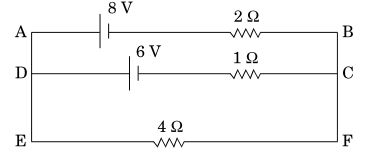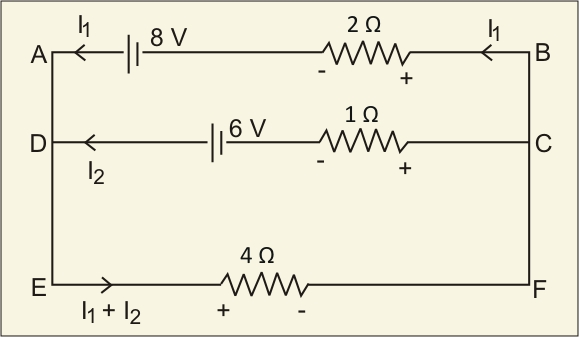# (a) State the working principle of a meter bridge used to measure an unknown resistance.(b) Give reason:        (i) why the connections between the resistors in a meter bridge are made of thick copper strips.        (ii) why is it generally preferred to obtain the balance length near the mid - point of bridge wire.(c) Calculate the potential difference across theresistor in the given electrical circuit, using Kirchhoff's rules.(a)Meter bridge is the simplest practical application of the Wheatstone bridge that's used to measure an unknown resistance.

Principle of meter bridge:-

It's working is based on the principle of Wheatstone bridge.

Working of a meter bridge:-

On moving the jockey along the wire, the galvanometer shows no deflection, that's said to be the balanced condition of the Wheatstone bridge.

If P and Q are the resistance of parts AB and BC of the wire, then for the balanced condition of the bridge, we haveif the total length of wire AC = 100cm and AB = lcm then BC = (100-l)cm. Since the bridge wire is of uniform cross-section, therefore,

the resistance of the wire of length l

ororwhere , s is the resistance per unit length of wire. Hence,Knowing I and R, 'S' can be determined as unknown resistance.

(b) (i) Since the resistivity of the copper wire is very low, that's why the connections between the rsistors in a meter bridge are made of thick copper strips.

So, the connections are thick, hence the are become very large and the resistance of wire becomes almost negligible.

(ii) As to increase the sensitivity of the meter bridge, it is generally preffered to obtain the balance point in the middle of the meter bridge.

(c)To determine the current,...(i)

For loop DEFCD........(ii)

From 1From equation (i)The net current acrossresistor is given by,

SoHence, V=IR

here R =4 then,## Most Viewed Questions

### Preparation Products

##### Knockout CUET (Physics, Chemistry and Mathematics)

Complete Study Material based on Class 12th Syllabus, 10000+ Question Bank, Unlimited Chapter-Wise and Subject-Wise Mock Tests, Study Improvement Plan.

₹ 7999/- ₹ 4999/-
##### Knockout CUET (Physics, Chemistry and Biology)

Complete Study Material based on Class 12th Syllabus, 10000+ Question Bank, Unlimited Chapter-Wise and Subject-Wise Mock Tests, Study Improvement Plan.

₹ 7999/- ₹ 4999/-
##### Knockout JEE Main (Six Month Subscription)

- AI Coach Study Modules, - Unlimited Mock Tests, - Study Improvement Plan.

₹ 9999/- ₹ 8499/-
##### Knockout JEE Main (Nine Month Subscription)

- AI Coach Study Modules, - Unlimited Mock Tests, - Study Improvement Plan.

₹ 13999/- ₹ 12499/-